# Dive Into Principal Component Analysis

## Introduction to PCA

Principal Component Analysis (PCA), is a linear dimension reduction method, which represents high dimensional data with low dimensional code.

If we can find a linear manifold in a high dimensional space, we can project the data onto this manifold, and just represent where it is on the manifold. Given a data matrix X, PCA is usually performed in the following steps:

1. Calculate the eigenvalues and eigenvectors for the Xᵀ X.
2. Select the top k eigenvalues, and the eigenvectors corresponding to these eigenvalues will be the principal components to choose.
3. Project the data onto the manifold formed by these k components.

A typical explanation of PCA:

What PCA does is taking m-dimensional data and finds the k orthogonal directions (also called principal components) in which the data have the most variance. These k principal components form a lower-dimensional subspace, we can then represent a m-dimensional datapoint by its projections onto the kprincipal directions. This loses all information about where the datapoint is located in the remaining orthogonal directions. However, since in PCA, we find the k principal components that have the largest variance explained, those left-out directions don't cover a lot of variance, we haven't lost much information.

We see the term of “variance” shows up a lot in the typical explanation of PCA. We can understand this intuitively, since we always want to choose the directions where data can spread out, which are the directions of larger “variance.” However, the resources convincing us of this point mathematically are limited.

## Purpose of This Blog Post

The purpose of this blog post is as follows:

• Provide a concise mathematical proof on why PCA is finding the directions where data have largest variance.
• Give readers a better knowledge of the math behind PCA, which helps to apply the method in a correct way and come up with better similar methods.
• Discuss whether centering data is necessary or not in doing PCA.
• Links PCA to a well-known algorithm in machine learning, called AutoEncoder.

## Reconstruction Error and PCA

Say X is our original data matrix, V is a matrix whose columns are orthonormal (Vᵀ V = I ). We define Y as: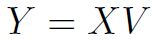(1)

V  is the matrix used for doing projection, andY  is the projected matrix. The dimensions of these matrices are as follows: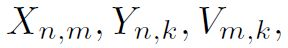with n being the number of samples, being the number of features, and k being the number of features in the projected space. When we try to project this back to the original space, we can do: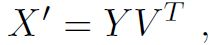(2)

we call X'  the reconstructed matrix. Combining (1) and (2), we have: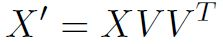(3)

The information loss (we call this reconstruction error) through this process is: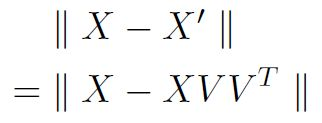(4)

This reconstruction error is what we want to minimize when we choose a projection matrix, since we always want to minimize the information loss, and this is the fundamental metric to evaluate whether a projection algorithm is good or not.

Proposition 1. Minimizing reconstruction error is equivalent to maximizing trace(Vᵀ Xᵀ XV ).

Proof.
Let(5)

It’s easy to show that: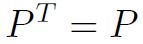(6)

and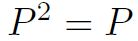(7)

Then (4) becomes: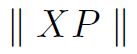(8)

Then we have: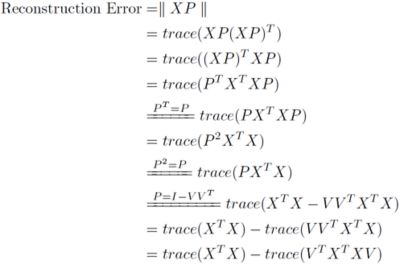(9)

We want to minimize (9), since X is fixed, this is equivalent to finding the V that maximizes trace (Vᵀ Xᵀ XV), and we complete the proof.

Now, we will prove the following statement: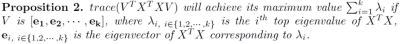Proof.
First, we note that XᵀX can be written as: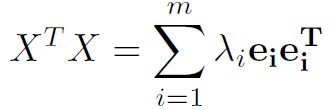(10)

Let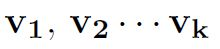be the column vectors of V, also we have: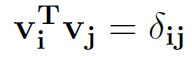Then: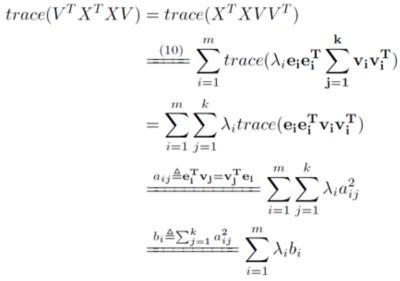(11)

Note that: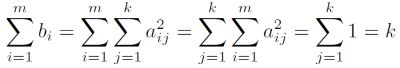(12)

and also: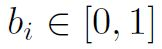(13)

From (12) and (13), we will have (proof omitted here):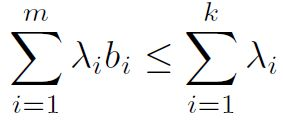It is obvious that when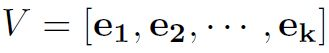we have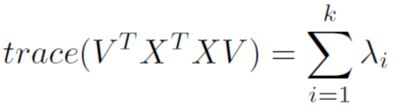thus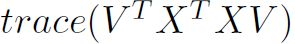achieves its maximum value when V is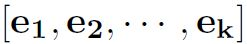To this point, we have completed the proof for Proposition 1 and Proposition 2; with them combined, we can reach the conclusion: to achieve the minimum reconstruction error, we can use a projection matrix that's composed of Xᵀ X's eigenvectors which correspond to Xᵀ X's top eigenvalues.

This is exactly what PCA does. Thus, PCA is constructed to choose the k components that can minimize the reconstruction error.

Notice that in this whole process, we never center the data. So even if we don't center the data, PCA still can give us the components which can minimize the reconstruction error.

## Variance and PCA

What will the situation be if we centered the data then?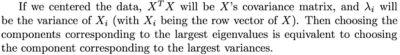To this point, we mathematically showed why PCA on centered data is trying to find the k components on which the data have the most variance.

So, choosing the components with largest data variance happens to be an interpretation of PCA when the data is centered. When the data is not centered, we lose this interpretation.

However, we can still perform the PCA steps, and the projection matrix found that way can also minimize the reconstruction error defined in (4).

## Relationship to AutoEncoder

The term “Reconstruction Error” often reminds us of a well-known algorithm called "Autoencoder." Actually, we indeed can perform PCA with an “Autoencoder.”

If we train an Autoencoder network where the hidden layer and output layer are linear, so that it will learn hidden units that are a linear function of the data and minimize the squared reconstruction error, the hidden units will span the same space as the first components found by PCA.

These k components tend to have equal variances, not like in PCA, they have the largest variances in large to small ordering. However, since they span the same space as the k components PCA finds, the reconstruction error will actually be the same as what PCA gets.

We can use gradient descent or any optimization algorithm to find this hidden code. To this point, PCA is reduced to be the same as other machine learning algorithms in terms of the fact that they are all trying to solve an optimization problem.

We can see this puts a restriction on the AutoEncoder network that the network has to be linear. If we make this nonlinear, then it will become the normal AutoEncoder, and can find encodings that are nonlinear combinations of the input. Thus, we can use AutoEncoder to perform non-linear dimension reduction.

## Conclusion

• Performing PCA is to find the low-rank approximation that minimizes the reconstruction error defined in (4).
• Finding principal components where data have the most variances is an interpretation of PCA when the data matrix is centered.
• AutoEncoder, after applying some restrictions, can be used to do PCA. Without the restriction, AutoEncoder can be used to perform non-linear dimension reduction.